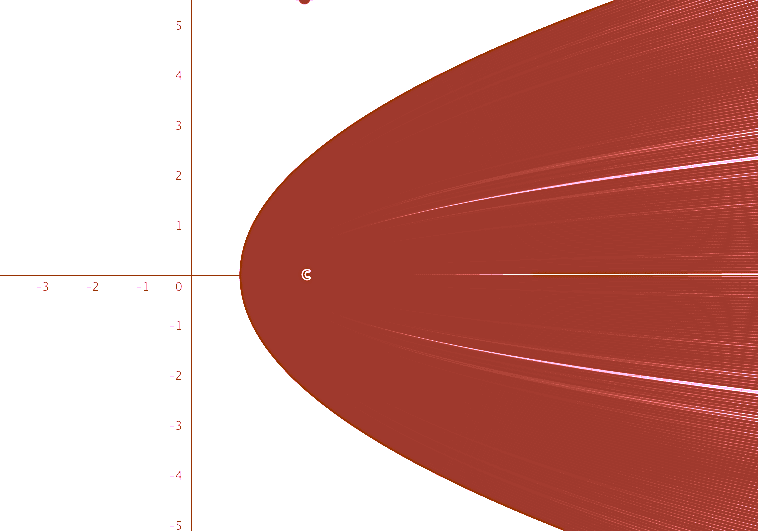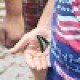How Cheenta works to ensure student success?
Explore the Back-Story

# I.S.I B.Stat Paper 2019 Subjective| problems & solutionsHere, you will find all the questions of ISI Entrance Paper 2019 from Indian Statistical Institute's B.Stat Entrance. You will also get the solutions soon of all the previous year problems.

Problem 1:

Prove that the positive integers $n$ that cannot be written as a sum of $r$ consecutive positive integers, with $r>1$, are of the form $n=2^{l}$ for some $l \geq 0$.

Problem 2:

Let $f:(0, \infty) \rightarrow \mathbb{R}$ be defined by
$$f(x)=\lim _{n \rightarrow \infty} \cos ^{n}\left(\frac{1}{n^{x}}\right)$$
(a) Show that $f$ has exactly one point of discontinuity.
(b) Evaluate $f$ at its point of discontinuity.

Problem 3:

Let $\Omega={z=x+i y \in \mathbf{C}:|y| \leq 1} .$ If $f(z)=z^{2}+2,$ then draw a
sketch of
$$f(\Omega)={f(z): z \in \Omega}$$

Problem 4:

Let $f: \mathbf{R} \rightarrow \mathbf{R}$ be a twice differentiable function such that
$$\frac{1}{2 y} \int_{x-y}^{x+y} f(t) d t=f(x), \quad \text { for all } x \in \mathbf{R}, y>0$$
Show that there exist $a, b \in \mathbf{R}$ such that $f(x)=a x+b$ for all $x \in \mathbb{R}$

Problem 5:

A subset $S$ of the plane is called convex if given any two points $x$ and $y$ in $S,$ the line segment joining $x$ and $y$ is contained in $S .$ A quadrilateral is called convex if the region enclosed by the edges of the quadrilateral is a convex set.

Show that given a convex quadrilateral $Q$ of area $1,$ there is a rectangle $R$ of area 2 such that $Q$ can be drawn inside $R$.

Problem 6:

For all natural numbers $n$, let
$$A_{n}=\sqrt{2-\sqrt{2+\sqrt{2+\cdots+\sqrt{2}}}}(n \text { many radicals })$$
(a) Show that for $n \geq 2$.
$$A_{n}=2 \sin \frac{\pi}{2^{n+1}}$$
(b) Hence, or otherwise, evaluate the limit
$\lim_{n \to \infty} 2^{n}A_{n}$

Problem 7:

Let $f$ be a polynomial with integer coefficients. Define
$$a_{1}=f(0), a_{2}=f\left(a_{1}\right)=f(f(0))$$
and
$$a_{n}=f\left(a_{n-1}\right) \quad \text { for } n \geq 3$$
If there exists a natural number $k \geq 3$ such that $a_{k}=0,$ then prove that either $a_{1}=0$ or $a_{2}=0$.

Problem 8:

Consider the following subsets of the plane:
$C_{1}=\{(x, y): x>0, y=\frac{1}{x}\}$
and
$C_{2}=\{(x, y): x<0, y=-1+\frac{1}{x}\}$
Given any two points $P=(x, y)$ and $Q=(u, v)$ of the plane, their distance $d(P, Q)$ is defined by
$$d(P, Q)=\sqrt{(x-u)^{2}+(y-v)^{2}}$$
Show that there exists a unique choice of points $P_{0} \in C_{1}$ and $Q_{0} \in C_{2}$ such that
$d\left(P_{0}, Q_{0}\right) \leq d(P, Q)$ for all $P \in C_{1}$ and $Q \in C_{2}$

## Some useful Links:

Here, you will find all the questions of ISI Entrance Paper 2019 from Indian Statistical Institute's B.Stat Entrance. You will also get the solutions soon of all the previous year problems.

Problem 1:

Prove that the positive integers $n$ that cannot be written as a sum of $r$ consecutive positive integers, with $r>1$, are of the form $n=2^{l}$ for some $l \geq 0$.

Problem 2:

Let $f:(0, \infty) \rightarrow \mathbb{R}$ be defined by
$$f(x)=\lim _{n \rightarrow \infty} \cos ^{n}\left(\frac{1}{n^{x}}\right)$$
(a) Show that $f$ has exactly one point of discontinuity.
(b) Evaluate $f$ at its point of discontinuity.

Problem 3:

Let $\Omega={z=x+i y \in \mathbf{C}:|y| \leq 1} .$ If $f(z)=z^{2}+2,$ then draw a
sketch of
$$f(\Omega)={f(z): z \in \Omega}$$

Problem 4:

Let $f: \mathbf{R} \rightarrow \mathbf{R}$ be a twice differentiable function such that
$$\frac{1}{2 y} \int_{x-y}^{x+y} f(t) d t=f(x), \quad \text { for all } x \in \mathbf{R}, y>0$$
Show that there exist $a, b \in \mathbf{R}$ such that $f(x)=a x+b$ for all $x \in \mathbb{R}$

Problem 5:

A subset $S$ of the plane is called convex if given any two points $x$ and $y$ in $S,$ the line segment joining $x$ and $y$ is contained in $S .$ A quadrilateral is called convex if the region enclosed by the edges of the quadrilateral is a convex set.

Show that given a convex quadrilateral $Q$ of area $1,$ there is a rectangle $R$ of area 2 such that $Q$ can be drawn inside $R$.

Problem 6:

For all natural numbers $n$, let
$$A_{n}=\sqrt{2-\sqrt{2+\sqrt{2+\cdots+\sqrt{2}}}}(n \text { many radicals })$$
(a) Show that for $n \geq 2$.
$$A_{n}=2 \sin \frac{\pi}{2^{n+1}}$$
(b) Hence, or otherwise, evaluate the limit
$\lim_{n \to \infty} 2^{n}A_{n}$

Problem 7:

Let $f$ be a polynomial with integer coefficients. Define
$$a_{1}=f(0), a_{2}=f\left(a_{1}\right)=f(f(0))$$
and
$$a_{n}=f\left(a_{n-1}\right) \quad \text { for } n \geq 3$$
If there exists a natural number $k \geq 3$ such that $a_{k}=0,$ then prove that either $a_{1}=0$ or $a_{2}=0$.

Problem 8:

Consider the following subsets of the plane:
$C_{1}=\{(x, y): x>0, y=\frac{1}{x}\}$
and
$C_{2}=\{(x, y): x<0, y=-1+\frac{1}{x}\}$
Given any two points $P=(x, y)$ and $Q=(u, v)$ of the plane, their distance $d(P, Q)$ is defined by
$$d(P, Q)=\sqrt{(x-u)^{2}+(y-v)^{2}}$$
Show that there exists a unique choice of points $P_{0} \in C_{1}$ and $Q_{0} \in C_{2}$ such that
$d\left(P_{0}, Q_{0}\right) \leq d(P, Q)$ for all $P \in C_{1}$ and $Q \in C_{2}$

## Some useful Links:

This site uses Akismet to reduce spam. Learn how your comment data is processed.

### 37 comments on “I.S.I B.Stat Paper 2019 Subjective| problems & solutions”

1.Ankush says:

In the third question the area of the resultant polygon is 2/3rd of the area of the triangle
Area = 2/3 x 9√3/2
= 3√3
The answer is option d

1.Ashani Dasgupta says:

Ankush double checking (possibly you are right), isn’t area of the triangle rt3/4 *(3^2)? 2/3rd of that is ..

1.Ankush says:

Okay I made a mistake at such a silly thing. Thanks for correcting it sir

2.Mrityunjay Shukla says:

16th Question the right answer should be 33 , it's not such an easy case of PHP as it seems like, for that we have to calculate
Number of Student who visited 5 days- 5C5=1
Number of Student who visited 4 days- 5C4=5
Number of Student who visited 3 days- 5C3=10
Number of Student who visited 2 days- 5C2=10
Number of Student who visited 1 day - 5C1=5
Number of Student who visited 0 day - 5C0=1
Total 32 cases, now applying Pigeon Hole Principle we need 33 students

1.Ashani Dasgupta says:

Yes. Fixed it! Thank you.

3.Nilava Metya says:

For objective #16, if we represent the days visited as a YES/NO tuple of (a1,a2,...,a5), then for each student, 2^5=32 such tuples are possible. So, there are 32 boxes and so the minimum number of students needed to guarantee the condition (in question) has to be 33. So answer should be (C)33.

1.Ashani Dasgupta says:

Yes. We just fixed that option. Thank you.

4.Sayantan Saha says:

26 should be option A.
Are the options of 18 correct

1.Ashani Dasgupta says:

Check that n=17 works for Problem 26
Yes, the options of 18 are correct.

1.Nilabh Kalita says:

18's correct option should be A.Let's assume we sent two children together, one children alone and both adults separately then we found that the boat has to cross the river 9 times

2.Prashasti Agarwal says:

In ques. 29 graph of x^2 and e^x intersect at 2 points so ans. Should be 2

1.Ashani Dasgupta says:

Trying drawing the graphs using GeoGebra and check how many times they intersect.

5.Prashasti Agarwal says:

In ques. 19, for adults it would be 4 times(2 for each) and for 2 children 2 times and as it is written no adult so 'max'... So for last 1 children, 2 times so total 4+2+2=8.. Sir please tell options are true.

1.Ashani Dasgupta says:

Remember, someone needs to row the boat back. It cannot come back empty to take more passengers.

6.himani agrawal says:

23
option B
as 79, 78, 77, 74, 73, 69
are not possible hence 100 - 6 = 94

1.Ashani Dasgupta says:

Yes that is right. That you for the input.

1.varun agrawal says:

total numbers are 101 not 100

7.himani agrawal says:

10th question
isn't that 1 is a finite number hence option D should also be correct

1.Ashani Dasgupta says:

No because D restricts the angles to certain values. But there are solutions for all angles.

8.varun agrawal says:

in 10th question 1 is also a finite number
hence option D should also be correct

1.Ashani Dasgupta says:

No because D restricts the angles to certain values. But there are solutions for all angles.

1.varun agrawal says:

they have told m are integer's' and n is not equal to zero which includes all real numbers
they haven't specifically said that values n are also are integers

1.Ashani Dasgupta says:

I hope that works out. However, usually, when mathematicians say integers m and n not 0, they usually mean both m and n are integers and n is not 0. Anyway, maybe your answer is correct. We have posted unofficial options.

2.Arkaprava ray says:

9.Rudransh Pratap Singh says:

for the 11th march to 15th march question,why should we consider not swimming on any dates as a possibility ?The empty set should not be considered,since we are asked to figure out whether they 'swim' on the same dates.Not swimming at all shouldn't be an option.

1.Mrityunjay Shukla says:

A child can be absent on all days and still be a student of the class.

10.Aranyak says:

I gave ISI BMath 2019 and scored 93 in UGA, And in UGB I attempted total 6 questions,3 questions correctly with 2 being partially answered and one being fully wrong, I am OBC too, Will I be called for the interview?

1.Ashani Dasgupta says:

That seems possible.

1.Aranyak says:

but the one i did wrong was the first question.... is it something dangerous.... i mean about the impression to the checker?

11.Md Zuhair says:

How is everyone's marks in UGA 2019? (acc. to official answer key) How much are you scoring?

1.SAANDEEP says:

99

12.Md Zuhair says:

What is the expected cutoff of ISI BMath 2019? (Like 2UGA+9UGB >= How much??)

13.Nilabh Kalita says:

Correct option of question no. 19 should be D. We can get 3 also there from the determinant.

14.Pranab Panda says:

HOW DID YOU GET THE SOLUTION FOR Q.26 ?

I COULD NOT FIND ANY SOLUTION SO I THINK ANSWER WILL BE 0 i.e (A).

IF YOU GET 1 SOLUTION STISFYING THE CONDITION THEN FOR WHAT VALUE OF n IS IT FOR?

1.Atul says:

n = 17

15.SAANDEEP says:

respected sir, the solutions of all the subjective questions are not uploaded.

16.samprit chakraborty says:

sir #16 will be (b) 1
as the slope of e^x is always > any polynomial function.it only cut once in the 2nd quadrant.

### Knowledge Partner

Cheenta is a knowledge partner of Aditya Birla Education Academy